Courses

# Basics Of Refrigerating Machine - 1

## 10 Questions MCQ Test Mock Test Series - Mechanical Engineering (ME) for GATE 2020 | Basics Of Refrigerating Machine - 1

Description
This mock test of Basics Of Refrigerating Machine - 1 for Mechanical Engineering helps you for every Mechanical Engineering entrance exam. This contains 10 Multiple Choice Questions for Mechanical Engineering Basics Of Refrigerating Machine - 1 (mcq) to study with solutions a complete question bank. The solved questions answers in this Basics Of Refrigerating Machine - 1 quiz give you a good mix of easy questions and tough questions. Mechanical Engineering students definitely take this Basics Of Refrigerating Machine - 1 exercise for a better result in the exam. You can find other Basics Of Refrigerating Machine - 1 extra questions, long questions & short questions for Mechanical Engineering on EduRev as well by searching above.
QUESTION: 1

Solution:
QUESTION: 2

Solution:
QUESTION: 3

### The domestic refrigerator has.a refrigeration load of the order of

Solution:
QUESTION: 4

Round the clock cooling of an apartment having a load of 450 MJ/day requires an air-conditioning plant of capacity about

Solution: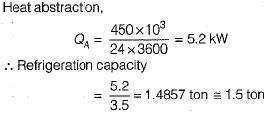QUESTION: 5

One tonne refrigerator machine means that

Solution:
QUESTION: 6

Match the List-I (Application) with List-ll (Refrigeration temperature)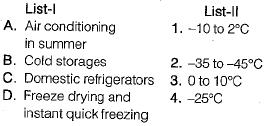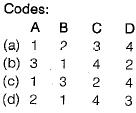Solution:
QUESTION: 7

The coefficient of performance of a domestic refrigerator is

Solution:
QUESTION: 8

Which one of the following is correct relation between (COP)HP and (COP)R

Solution:

Relation between coefficient of performance of heat pump and refrigerator: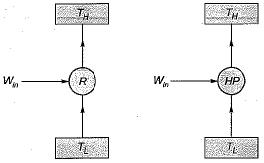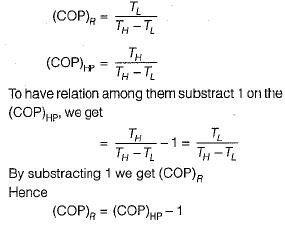QUESTION: 9

Horse power and kW required per tonne of refrigeration is expressed as

Solution: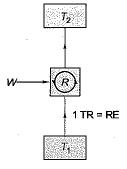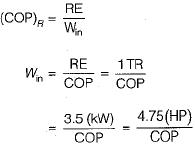QUESTION: 10

One tonnes of refrigeration is equal to

Solution:

1 T.R. - 3.5 kW = 210 kJ/min